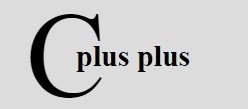# C++ References

« Previous Tutorial Next Tutorial »

Reference is used to operate on any existing variable's memory address inside C++ program:

## Create Reference in C++

To create a reference variable in C++. Let's take a look at the following example. Here there is a definition of a simple variable.

`int i = 17;`

We can declare reference variables for i like this:

`int& ir = i;`

Read the & in these declarations as reference.

## C++ References Example

Here is an example program demonstrating the use of references on int and double:

```/* C++ References */

#include<iostream.h>
#include<conio.h>
void main()
{
clrscr();
int i;
double d;
int &ir = i;
double &dr = d;
cout<<"Enter any two number (integer and double) : ";
cin>>i>>d;
cout<<"\nValue of i (simple) : "<<i<<"\n";
cout << "Value of i (reference) : "<<ir<<"\n";
cout<<"Value of d (simple) : "<<d<<"\n";
cout<<"Value of d (reference) : "<<dr<<"\n";
getch();
}```

Here is the sample run of the above C++ program:

C++ Online Test

« Previous Tutorial Next Tutorial »